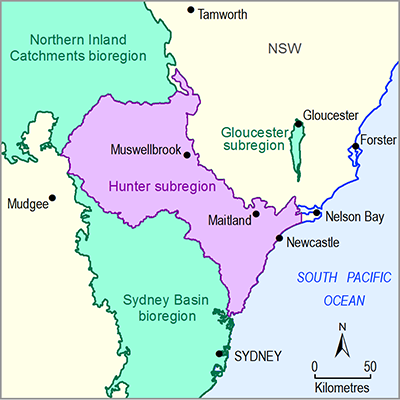# 2.6.2.6.1 Hydraulic properties

Page 31 of 56

The groundwater model needs to define porosity and vertical and horizontal hydraulic conductivity for every element in the model mesh. These properties might be expected to vary with lithology or geology. An analysis of 577 hydraulic conductivity measurements from the Hunter subregion found little to no correlation between lithology and hydraulic conductivity, nor between geologic layer and hydraulic conductivity (see Section 2.1.3 in companion product 2.1-2.2 for the Hunter subregion (Herron et al., 2018)). However, there was a weak correlation with depth (see Figure 26 in Herron et al. (2018)). Companion submethodology M07 for groundwater modelling (Crosbie et al., 2016) proposes the use of a simple parameterisation of hydrostratigraphy in bioregional assessment (BA) groundwater model layers that treats the layers as homogeneous, but varies hydraulic properties with depth. In the absence of a good basis for varying hydraulic properties by lithology or geology, the parameterisation of the Hunter subregion groundwater model adopts this approach.

As described in Section 2.6.2.3.3.1, every point in the model domain can be defined in terms of a layer number (N = 1 to 11), a lithology (L = 1 to 7) and a depth, d, below the model topography. The horizontal hydraulic conductivity, Kh, the vertical hydraulic conductivity, Kv, and the porosity, Φ, are assumed to be of the form:(10)(11)(12)

where a is the exponential decay coefficient which varies each parameter with depth.

Reference horizontal conductivities, porosities and decay parameters are defined in Table 6, and were obtained by appropriately averaging data from Figure 26 and Figure 31 of Herron et al. (2018). It is not appropriate to directly use the raw conductivity data presented in Figure 26 in the groundwater model. This is because the measured data pertain to samples on the scale of centimetres (for lab measurements) to a few tens of metres (in-situ measurements). The regional groundwater model has a best resolution of 500 m, so some ‘upscaling’ of the raw data is necessary. Consider the problem of prescribing a suitable conductivity to a 500 m region of the groundwater model. Typically, there will be some regions of low conductivity within that region but there will also be regions of high conductivity, and water will flow preferentially through those highly conductive regions, almost entirely bypassing the regions of low conductivity. Therefore, the 500 m region will have an effective conductivity that is closer to the high conductivities shown in Figure 26. Of course, given the scatter of raw data in Figure 26, the conductivity of our 500 m region will be drawn from a probability distribution of conductivity values, but the mean of this probability distribution will be the (arithmetic) mean of the raw data. A classic review of this subject may be found in Renard and de Marsily (1997). This arithmetic mean is shown in Figure 26 as a function of depth, and leads to the value shown in Table 6.

Less data are available to constrain vertical conductivity. Aquitards typically occur as roughly horizontal layers of low conductivity in stratified geological systems such as the Hunter subregion. Such aquitards have little effect on horizontal water flow, since it flows relatively quickly through the surrounding aquifers, but have a greater effect on vertical flow, since the water must pass through the aquitard. This means that groundwater models typically use a vertical conductivity that is a small multiple of horizontal conductivity. A base multiplying factor of 0.1 is chosen in the Hunter groundwater model (and this is varied in the uncertainty analysis).

The alluvium layer (L = 7) is assumed to be more porous and conduct water more rapidly than the interburden layers (L = 1 to 6), reflecting its unconsolidated nature. Its thickness is represented as one finite element, which means at any point the conductivity of the alluvium is a single number and does not vary with depth. However because the thickness of the alluvium varies across the subregion, and conductivity is evaluated at the centroid of a 3D finite element, the effect of the decay parameter means a 20 m thick alluvium will have conductivity of 0.78 m/day, while a 10 m thick alluvium will have conductivity of 0.88 m/day. These differences are negligible. Thus the decay for the alluvium layer is unimportant since it is so thin in comparison with the overall model thickness.

Horizontal and vertical conductivities are assumed to decay at the same rate with depth.

Table 6 Reference hydraulic property values and decay parameters

Parameter

Units

Interburden layers

(1 to 6)

Alluvium layer

(7)

Horizontal hydraulic conductivity, Kh(L,N)

m/day

0.5

1.0

Vertical hydraulic conductivity, Kv(L,N)

m/day

0.05

1.0

Porosity, Φ(L,N)

dimensionless

0.1

0.2

Decay parameters, ah , av

m–1

0.025

0.025

Decay parameter, aΦ

m–1

0.01

0.01

In many groundwater models, the decay parameters ah, av, aΦ, and the functions Kh(L,N), Kv(L,N) and Φ(L,N) would be calibrated by observation with water heads. Here, however, these are all varied in the uncertainty analysis as described in Section 2.6.2.7.2

In the coal resource development pathway and baseline simulations, the horizontal and vertical conductivities are further enhanced above underground mines and below both open-cut and underground mines.

Last updated:
18 January 2019